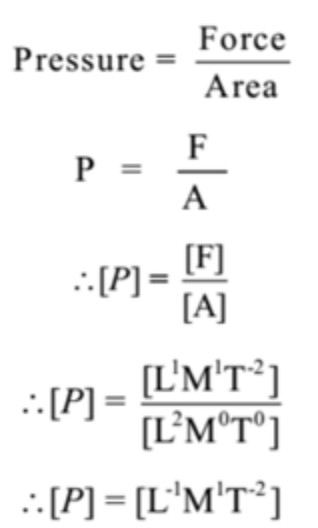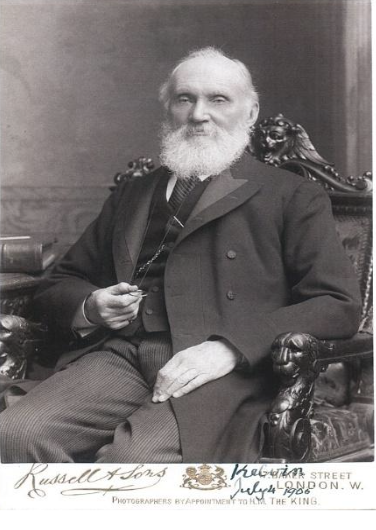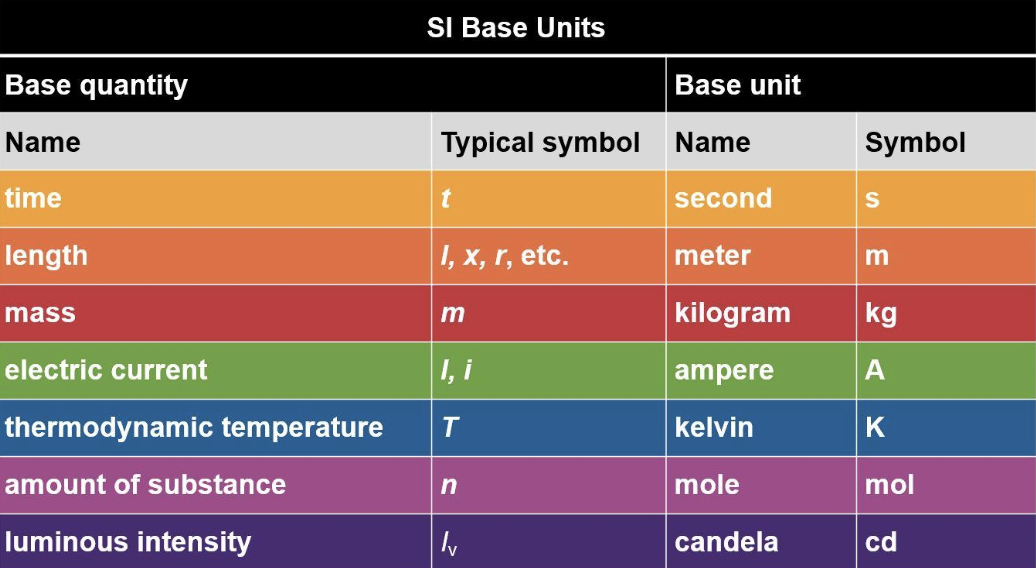# TL;DR Science: Quantities, Units and Dimensional AnalysisBy Philip A.
November 11, 2022 · 4 minute read

Physics

Biological Engineering

MathematicsPhysics is all about observing natural phenomena. We can observe different aspects of physical phenomena. These aspects are called physical quantities. Some examples are mass, force, temperature, length etc.  Systematic observation of these quantities in different phenomena is essential in developing laws and building models that predict how they change.

When observing a physical phenomenon, the first step is to notice a pattern emerge between two quantities. For example, it is empirically understood that if you push a massive object with a greater force, the more it will accelerate. This tells us that there is a linear relationship between force and acceleration. This kind of analysis is called qualitative in that it doesn’t tell us exactly how much the two quantities are related. It only describes the kind of relation(proportional, inversely proportional etc.).

To help with this kind of analysis there exists a method called dimensional analysis. Each quantity has a dimension. In classical physics, we define three base dimensions: length, mass, and time. All quantities that are defined from the previously mentioned dimensions are called derived quantities whereas the base dimensions describe elementary quantities. For example, speed is defined as the distance an object traces in a certain timeframe(v = x/t) so speed has dimensions of length over time. Dimensional analysis tells us that each qualitative relation has to obey dimensional homogeneity in other words the two sides of the equation need to have the same dimension.This turns out to be very useful when studying different physical phenomena. Its two main uses are: verifying the validity of a qualitative relation and predicting a qualitative relation given the quantities involved. Simply put, you can see if an equation is true based on the dimensions of the quantities on each side and you can predict the kind of relation(proportional, quadratic etc) between two quantities in a physical phenomenon.Although this might initially help us study a phenomenon, for an observation to be complete you have to measure the exact value of the quantities involved. Lord Kelvin was the first to state this: ‘When you can measure what you are speaking about, and express it in numbers, you know something about it; but when you cannot measure it, when you cannot express it in numbers, your knowledge is of a meagre and unsatisfactory kind.'

But, to measure the exact value of something we have to define what we are measuring it against. A pure number cannot in any instance describe a physical quantity because it is meaningless. All physical quantities have a dimension which corresponds to something physical and intuitive while numbers are abstract representations. (This can be understood by the following anecdote: How much water do you need? Just three).

The way to measure quantities exactly is by defining base units where all quantities are measured against these base units. The way to define such bases has changed in history and even in this century. But, in physics, the SI has been universally adopted where base units are the meter the second the kilogram etc. Presently the way we define all base units is by expressing them in terms of natural constants like the speed of light.Source: NIST Special Publication 330:2019, Table 2

Having established base units we can confidently measure quantities. These measurements are of paramount importance for physics to have any sort of predictive power. You don’t want to know whether heat transfer is linearly proportional to time but how much energy in joules you need to cook a chicken of mass m in kg. Physical equations give us such exact predictions.

### TL;DR

Physics is the study of physical phenomena. We can observe different aspects of physical phenomena. These aspects are called physical quantities. In the study of physical phenomena, scientists come up with relations that combine different physical quantities. When talking about the kind of relation between two of these we define what a dimension is and we use dimensional analysis. On the other hand, when talking about predicting an exact value we have to define systems of measurement and units. These two concepts compactly describe the study of simple classical phenomena and are of paramount importance to physics.

##### Sources:
1. Serway, Raymond A. Physics for Scientists & Engineers with Modern Physics. Philadelphia: Saunders College Pub., 19861983
2. Physics World Magazine, In praise of Lord Kelvin